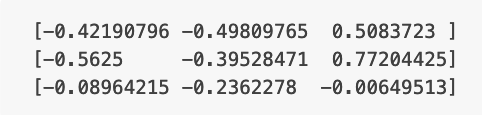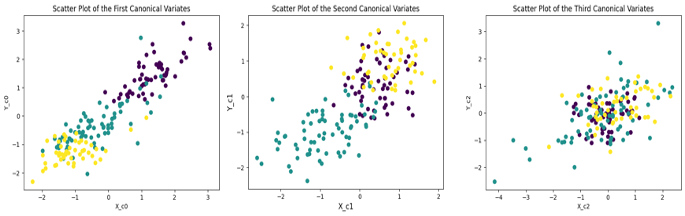### Exploring Canonical Correlation Analysis (CCA): Uncovering Hidden Relationships

Canonical Correlation Analysis (CCA) is a statistical technique that enables us to uncover hidden associations between two sets of variables. Whether it's in the fields of psychology, economics, genetics, marketing or machine learning, CCA proves to be a powerful tool for gaining valuable insights. In this blog post, we will try to understand CCA. But first let’s take a look at two sets of observations, X and Y, shown below. These two sets of observations are made on the same set of objects and each observation represents a different variable.

Let’s calculate pairwise correlation between the column vectors of X and Y. The resulting correlation values should give us some insight between the two sets of measurements. These values are shown below where the entry at (i,j) represents the correlation between the i-th column of X and the j-th column of Y.The correlation values show moderate to almost no correlation between the columns of the two datasets except a relatively higher correlation between the second column of X and the third column of Y.

### Is There a Hidden Relationship?

It looks like there is not much of a relationship between X and Y. But wait! Lets transform X and Y into one-dimensional arrays, a and b, using the vectors [-0.427 -0.576 0.696] and [0 0 1].

a = X[-0.427 -0.576 0.696]T

b = Y[0 0 1]T

Now, let's calculate the correlation between a and b. Wow! we get a correlation value of -0.999, meaning that the two projections of X and Y are very strongly correlated. In other words, there is a very strong hidden relationship present in our two sets of observations. An obvious question on your part at this stage is “how did you get the two vectors above used for getting a and b?” The answer to this is the canonical correlation analysis.

### What is Canonical Correlation Analysis?

Canonical correlation analysis is the problem of finding pairs of basis vectors for two sets of variables X and Y such that the correlation between the projections of the variables onto these basis vectors are mutually maximized. The number of pairs of such basis vectors is limited to the smallest dimensionality of X and Y. Assume $\bf{w}_x$ and $\bf{w}_y$ be the pair of basis vectors that projects X and Y into a and b given by $\bf a = X w{_x}$, and $\bf b = Y w{_y}$. The projections a and b are called the scores or the canonical variates. The correlation between the projections, after some algebraic manipulation, can be expressed as:

$\Large \rho = \frac{\bf{w}_{x}^T \bf{C}_{xy}\bf{w}_{y}}{\sqrt{\bf{w}_{x}^T \bf{C}_{xx}\bf{w}_{x}\bf{w}_{y}^T \bf{C}_{yy}\bf{w}_{y}}}$,

where Cxx, Cxy and Cyy  are three covariance matrices. The canonical correlations between X and Y are found by solving the eigenvalue equations

$\bf{C}_{xx}^{-1}\bf{C}_{xy}\bf{C}_{yy}^{-1}\bf{C}_{yx}\bf{w}_x = \rho^2 \bf{w}_x$

$\bf{C}_{yy}^{-1}\bf{C}_{yx}\bf{C}_{xx}^{-1}\bf{C}_{xy}\bf{w}_y = \rho^2 \bf{w}_y$

The eigenvalues in the above solution correspond to the squared canonical correlations and the corresponding eigenvectors yield the needed basis vectors. The number of non-zero solutions to these equations are limited to the smallest dimensionality of X and Y.

### CCA Example

Let’s take a look at an example using the wine dataset from the sklearn library. We will divide the13 features of the dataset into X and Y sets of observations. The class labels in our example will act as hidden or latent feature. First, we will load the data, split it into X and Y and perform feature normalization.

from sklearn.datasets import load_wine
import numpy as np
X = wine.data[:, :6]# Form X using first six features
Y = wine.data[:, 6:]# Form Y using the remaining seven features
# Perform feature normalization
from sklearn.preprocessing import StandardScaler
scaler = StandardScaler()
X = scaler.fit_transform(X)
Y = scaler.fit_transform(Y)

Next, we import CCA object and fit the data. After that we obtain the canonical variates. In the code below, we are calculating 3 projections, X_c and Y_c, each for X and Y.

from sklearn.cross_decomposition import CCA
cca = CCA(n_components=3)
cca.fit(X, Y)
X_c, Y_c = cca.transform(X, Y)
We can now calculate the canonical correlation coefficients to see what correlation values are obtained.
cca_corr = np.corrcoef(X_c.T, Y_c.T).diagonal(offset=3)
print(cca_corr)

[0.90293514 0.73015495 0.51667522]

The highest canonical correlation value is 0.9029, indicating a strong hidden relationship between the two sets of vectors. Let us now try to visualize whether these correlations have captured any hidden relationship or not. In the present example, the underlying latent information not available to CCA is the class-membership of different measurements in X and Y. To check this, I have plotted the scatter plots of the three sets of x-y canonical variates where each variate pair is colored using the class label not accessible to CCA. These plots are shown below. It is clear that the canonical variates associated with the highest correlation coefficient show the existence of three groups in the scatter plot. This means that CCA is able to discern the presence of a hidden variable that reflects the class membership of the different observations.### Summary

Canonical Correlation Analysis (CCA) is a valuable statistical technique that enables us to uncover hidden relationships between two sets of variables. By identifying the most significant patterns and correlations, CCA helps gain valuable insights with numerous potential applications. CCA can be also used for dimensionality reduction. In machine and deep learning, CCA has been used for cross-modal learning and cross-modal retrieval.

### LLaMA 2 and its Symbolic Regression Explanation

On July 17, a new family of AI models, LLaMA 2 was announced by Meta. LLaMA 2 is trained on a mix of publicly available data. According to Meta LLaMA 2 performs significantly better than the previous generation of LLaMA models. Two flavors of the model: LLaMA 2 and LLaMA 2-Chat, a model fine tuned for two-way conversations, were released. Each flavor further has three versions with the parameters ranging from 7 billions to 70 billions. Meta is also freely releasing the code and data behind the model for  researchers to build upon and improve the technology. There are several ways to access LLaMA 2 for development work; you can download it from HuggingFace or access it via Microsoft Azure or Amazon SageMaker . For those interested in interacting with the LLaMA 2-Chat version, you can do so by visiting llama2.ai , a chatbot model demo hosted by the venture capitalist Andreessen Horowitz. This is the route I took to interact with LLaMA 2-Chat. Since I was reading an excellent paper on

### Reinforcement Learning with Human Feedback: A Powerful Approach to AI Training

The unprecedented capabilities exhibited by the large language models (LLMs) such as ChatGPT and GPT-4 have created enormous excitement as well as concerns about the impact of AI on the society in near and far future. Behind the success of LLMs and AI in general lies among other techniques a learning approach called Reinforcement Learning with Human Feedback (RLHF). In this blog post, we will try to understand what RLHF is and why it offers a powerful approach to training AI models. However, before we do that, let's try to understand the concept of reinforcement learning (RL). What is Reinforcement Learning (RL)? RL, inspired by the principles of behavioral psychology, is a machine learning technique wherein the learner, called an agent , learns decision making by exploring an environment through a trial-and-error process to achieve its goal. Each action by the agent results in feedback in the form of a reward or punishment . While performing actions and receiving feedback, the a

### Low Rank Adaptation (LoRA): Enhancing Fine-Tuning of LLMs

Pre-trained large language models (LLMs) are being used for numerous natural language processing applications. These models perform well out of the box and are fine-tuned for any desired down-stream application. However, fine-tuning these models to adapt to specific tasks often poses challenges due to their large parameter sizes. To address this, a technique called Low Rank Adaptation (LoRA) has emerged, enabling efficient fine-tuning of LLMs. In this post, we will try to understand LoRA, and delve into its importance and application in fine-tuning LLMs. We will begin our journey by first looking at the concept of rank of a matrix, followed by a look at matrix factorization, and then to LoRA. Rank of a Matrix The rank of a matrix indicates the number of independent rows or column in the matrix. As an example, consider the following 4x4 matrix A: A = [[2, 4, 6, 8], [1, 3, 5, 7], [4, 8, 12, 16], [3, 9, 15, 21]] Looking at the first and third row of this matrix, we see that the third row